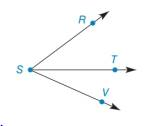Chapter 1.4, Problem 18E### Elementary Geometry for College St...

6th Edition
Daniel C. Alexander + 1 other
ISBN: 9781285195698

#### Solutions

Chapter
Section### Elementary Geometry for College St...

6th Edition
Daniel C. Alexander + 1 other
ISBN: 9781285195698
Textbook Problem
21 views

#Exercises 16 − 24 Given: m ∠ R S T = 2 x + 9 m ∠ T S V = 3 x − 2 m ∠ R S V = 67 ° Find: x

To determine

To find:

The value of x for the given information.

Explanation

Given:

mRST=2x+9mTSV=3x2mRSV=67°

The given figure is,

Definition:

If a point say M lies in the interior of PQR, then mPQM+mRQM=mPQR.

Calculation:

Consider the figure,

T is the interior point of the angle RSV.

### Still sussing out bartleby?

Check out a sample textbook solution.

See a sample solution

#### The Solution to Your Study Problems

Bartleby provides explanations to thousands of textbook problems written by our experts, many with advanced degrees!

Get Started

#### Write the sum in expanded form. 9. j=0n1(1)j

Single Variable Calculus: Early Transcendentals, Volume I

#### Simplify the expressions in Exercises 97106. x3/2x5/2

Finite Mathematics and Applied Calculus (MindTap Course List)

#### In Exercises 107-120, factor each expression completely. 116. (x + y)2 1

Applied Calculus for the Managerial, Life, and Social Sciences: A Brief Approach